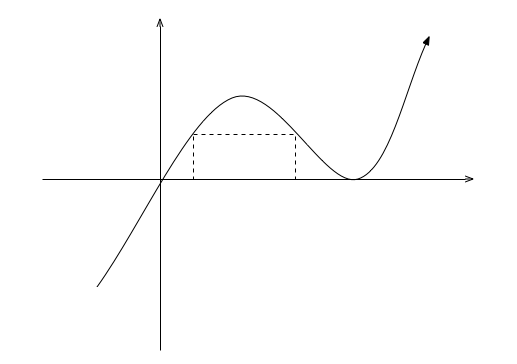# Find maximum area of rectangle

Calculus Level 3

A rectangle's bottom is at $y=0$while its top corners are on the curve $y=x{ (x-1) }^{ 2 }$ between $x=0$ and $x=1$. The maximum area of this rectangle can be expressed as

$\dfrac { a\sqrt { a } -b }{ c\sqrt { d } }$

where $a$ and $d$ are prime numbers. What is the sum $a+b+c+d?$

(Don't count $a$ twice)

×

Problem Loading...

Note Loading...

Set Loading...